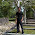## Sunday, February 13, 2011

### High Current Motor Controller Shield Schematic

Good news, folks! I've decided to post the schematics for the high current motor controller shield with some knowledge on how to use the VN2SP30 IC. The schematic is taken almost directly from the datasheet from ST. I left out the reverse voltage diode because I have a polarized connector, but I still managed to accidentally reverse voltage and fry it. The next rev will include reverse voltage protection.

Here is some sample code to run the motor controller. Disclaimer: This code was written in minutes and may not be the best code in the world

#### Example Sketch for the Motor Controller Shield

```/*
High current motor controller shield example sketch
ilektron-x.blogspot.com
*/
const int pwm1Pin = 5;   // the pin that the pwm1 is attached to
const int pwm2Pin = 6;   // the pin that the pwm2 is attached to
const int ina1Pin = 7;   // the pin that the ina1 is attached to
const int inb1Pin = 8;   // the pin that the inb1 is attached to
const int ina2Pin = 9;   // the pin that the ina2 is attached to
const int inb2Pin = 10;  // the pin that the inb2 is attached to

#define timestep 20
#define kscale 11370     // Calculated from datasheet, used to convert ADC reading to current
#define res 1500         // Current sense resistor value

void setup()
{
// initialize the serial communication:
Serial.begin(57600);

// initialize the motor controller pins as an output:
pinMode(pwm1Pin, OUTPUT);
pinMode(pwm2Pin, OUTPUT);
pinMode(ina1Pin, OUTPUT);
pinMode(inb1Pin, OUTPUT);
pinMode(ina2Pin, OUTPUT);
pinMode(inb2Pin, OUTPUT);

// Make sure everything is low, set up the motors to spin in opposite directions
digitalWrite(pwm1Pin, LOW);
digitalWrite(pwm2Pin, LOW);
digitalWrite(ina1Pin, HIGH);
digitalWrite(inb1Pin, LOW);
digitalWrite(ina2Pin, HIGH);
digitalWrite(inb2Pin, LOW);
}

void loop() {
static byte speed1 = 0;
byte rxdata = 0;
byte cs1 = 0;
static int countdown = timestep;

static long current1 = 0;
static long current2 = 0;
float cval1 = 0;
float cval2 = 0;

// check if data has been sent from the computer:
if (Serial.available()) {
// read the most recent byte (which will be from 0 to 255):

// 'u' key press ups speed, 'd' decreases it. 0-9 set the speed to 0-90% of speed
switch (rxdata)
{
case 'u':
speed1 = (speed1 < 250) ? speed1 + 5: 255;
break;
case 'd':
speed1 = (speed1 > 5) ? speed1 - 5: 0;
break;
case '0':
case '1':
case '2':
case '3':
case '4':
case '5':
case '6':
case '7':
case '8':
case '9':
case ':':
speed1 = (255*(rxdata-'0')/10);
break;
default:
speed1 = 0;
break;
}

// Display what the new speed setting is
Serial.print('\n');
Serial.print('s');
Serial.print(speed1, DEC);

// set the motor speed:
analogWrite(pwm1Pin, speed1);
analogWrite(pwm2Pin, speed1);

}

delay(1);
if (!countdown) // Simple countdown timer to space out frequency of current reading output
{
// Calculate current for motor 1
Serial.print("M1:\0");
cval1 = current1;
cval1 = (cval1*kscale/timestep)/res*5/1024;
Serial.print(cval1, 3);
// Calculate current for motor 2
Serial.print("\tM2:\0");
cval2 = current2;
cval2 = (cval2*kscale/timestep)/res*5/1024;
Serial.print(cval2, 3);
Serial.print('\n');
countdown = timestep;
current1 = 0;
current2 = 0;
} else {
countdown--;
}
}```

1.2.